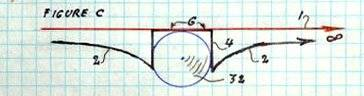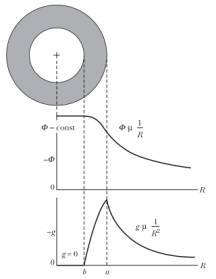# Flat spacetime in a gravity well

mfb
Mentor
He is simply of the mind that zero gravitational force should result in zero time dilation (just as it would be in the empty outer reaches of space).
As there is no point with zero gravitational force this is impossible to disprove experimentally. But any reasonable dependence on the force instead of the potential is completely inconsistent with measurements done in places with a non-zero force.

Gold Member
As there is no point with zero gravitational force this is impossible to disprove experimentally.
I think 'approaching zero' would surely suffice

But any reasonable dependence on the force instead of the potential is completely inconsistent with measurements done in places with a non-zero force.
I think that's part of what's got him going: the curve of gravitational force and the curve of time dilation look similar in all the places we've measured so far. He probably figures why would it stop being similar at the Earth's surface?

A.T.
He probably figures why would it stop being similar at the Earth's surface?
Because naive reasoning based on "looking similar" doesn't always work. Because a function and it's derivatives can "look similar" in some ranges, but not in others.

stevendaryl
Staff Emeritus
I think that's part of what's got him going: the curve of gravitational force and the curve of time dilation look similar in all the places we've measured so far. He probably figures why would it stop being similar at the Earth's surface?

I'm not sure what you mean by "similar", but the formula for proper time in terms of coordinate time involves the gravitational potential, rather than the gravitational force. The relation works whether you are above or below the Earth's surface.

The approximate relationship, good when gravitational fields are weak and velocities are low compared to the speed of light is (using Schwarzschild coordinates):

##\delta \tau = (1 + \frac{m \Phi_g - \frac{1}{2} m v^2}{mc^2}) \delta t##

where ##\Phi_g## is the gravitational potential, and ##v## is the velocity of the clock measuring ##\tau##. This approximate formula works above and below the surface.

Note: the potential ##\Phi_g## should be chosen so that ##\Phi_g \rightarrow 0## as ##r \rightarrow \infty##. For a different choice, the above formula needs to be adjusted slightly.

stevendaryl
Staff Emeritus
The approximate relationship, good when gravitational fields are weak and velocities are low compared to the speed of light is (using Schwarzschild coordinates):

##\delta \tau = (1 + \frac{m \Phi_g - \frac{1}{2} m v^2}{mc^2}) \delta t##

The approximate time-dilation factor, ##K \equiv 1 + \frac{m \Phi_g - \frac{1}{2} m v^2}{mc^2}##, depends on the gravitational potential, not the gravitational force. Since the force is the negative derivative of the potential, you have: ##\frac{\partial K}{\partial x} = \frac{1}{c^2} \frac{\partial \Phi_g}{\partial x} = - \frac{g}{c^2}##, where ##g## is the local acceleration due to gravity (in Schwarzschild coordinates---coordinate acceleration is a coordinate-dependent quantity). So ##g## being zero as it is at the center of the Earth doesn't mean that the time dilation is zero, it just means that the time dilation is approximately constant. So near the center of the Earth, there would not be a measurable location-dependence to time dilation. In contrast, on the surface of the Earth, where ##g## is nonzero, clocks that are higher run faster (as measured in Schwarzschild coordinates) tha clocks that are lower.

Gold Member
Since he has a naive understanding of GR in the first place, he's not going to follow the logic of equations.

But he has produced some diagrams, at my behest. Here is one of a hollow sphere, which has some unexplainable cusps and nigh-infinite slopes:I have started showing him the logical implications of his idea that lead to paradoxes. (Such as a watch on one wrist running at a dramatically different rate than the watch on the other wrist).

He has, predictably, begun to lose interest and wander away, mumbling about 'paradoxes' in Einsteinian models.

If anyone is interested in the dialogue, it is here:
poster's name is nebel.

#### Attachments

A.T.
Since he has a naive understanding of GR in the first place....
He should start with Newtonian gravity, and the difference between gravitational force and potential, as shown here:http://teacher.pas.rochester.edu/PHY235/LectureNotes/Chapter05/Chapter05.htm

Gravitational time dilation in GR is related to the potential (upper plot, note the inverted y-axis), and is maximal at the center where gravity in Newtonian sense is zero (lower plot).

#### Attachments

Last edited:
What about hovering at the midpoint between two identical, non-rotating black holes? My intuition at least tells me (if the black holes are close enough, even momentarily) that there will be significant time dilation, even though the net “gravitational force” will be 0 (let’s also say we’re relatively small enough that the tidal forces are negligible, just to make things simpler). But then again, my intuition also tells me time dilation will be greater at the center of the earth than at the surface, so who knows, this might be useless.

Jonathan Scott
Gold Member
By the shell theorem, the Newtonian potential outside a spherical body is the same as if the entire mass was concentrated at the central point, and the potential inside a spherical shell is the same everywhere. So as you go deeper, the contribution towards the potential of any shell you pass remains constant once you have passed it, and the potential due to the spherical shells that you haven't yet passed is steadily getting deeper due to the fact that you are getting closer to the middle.
[Edited to correct typo]

•stevendaryl
A.T.
What about hovering at the midpoint between two identical, non-rotating black holes? My intuition at least tells me (if the black holes are close enough, even momentarily) that there will be significant time dilation, even though the net “gravitational force” will be 0 (let’s also say we’re relatively small enough that the tidal forces are negligible, just to make things simpler). But then again, my intuition also tells me time dilation will be greater at the center of the earth than at the surface, so who knows, this might be useless.
Both is correct.

Gold Member
He should start with Newtonian gravity, and the difference between gravitational force and potential, as shown here:
Ah! Now we're getting somewhere.
How does gravitational potential manifest in Newtonian gravity?
What properties does it have that can be measured?

(So far, the only effect of GP I have been able to find is Einsteinian time dilation, thus my quandary.)

Jonathan Scott
Gold Member
In Newtonian gravity, the difference in potential (typically ##\phi = -Gm/r##) determines the difference in potential energy of an amount of mass in the relevant location compared with the reference location. In General Relativity, that is replaced with a fractional difference in energy due to time dilation, and to make it dimensionless it can be expressed in terms of change in energy for a given rest energy (typically ##\phi/c^2 = -Gm/rc^2##). So the relative fractional time dilation is the difference in the Newtonian potential in dimensionless units.

(Unless you are near a neutron star or worse, the potential is so tiny that the fractional changes in time rate effectively add linearly, as for Newtonian potentials).

Gold Member
In Newtonian gravity, the difference in potential (typically ##\phi = -Gm/r##) determines the difference in potential energy of an amount of mass in the relevant location compared with the reference location.
So, a body falling toward it would gain kinetic energy as it fell. But that happens anyway, as it approaches the mass (along the curve).
What measureable effect would it have once in the hollow sphere?

Oh. I think I see.
In my colleagues's graph:the implication of the cusp and vertical slope is that any mass would instantly get stuck to the inner surface of the sphere - where it has minimum potential energy. It would take, essentially escape velocity (i.e. enough velocity to get back to flat space at an infinite distance) to break away from the inner wall of the shell and head toward the centre.

#### Attachments

PeterDonis
Mentor
2020 Award
What about hovering at the midpoint between two identical, non-rotating black holes? My intuition at least tells me (if the black holes are close enough, even momentarily) that there will be significant time dilation, even though the net “gravitational force” will be 0

Your intuition is correct as far as it goes. However, there is a key difference between this situation and the situation of a single gravity well: this situation is not static. The holes will fall towards each other. That means that, strictly speaking, the concept of "gravitational time dilation" is not well-defined in this scenario.

It is possible to define "gravitational time dilation" for the specific case of the observer "hovering" at the midpoint between the holes--at least for the period before that observer crosses the horizon of the combined hole formed by the merger of the two holes falling together. This is because the observer "hovering" at the midpoint, while he remains outside the horizon of the combined hole, is at rest relative to an observer at infinity. But this definition does not generalize to other observers.

•Pencilvester
Your friend at the center of the Earth lives a daily life with normal time speed. He can cook boiled eggs in ten minutes of his watch and he will live 80 toghether with his old pendulum clock. There is no time dilation in his life.

Time dilation works when we compare his time and our Earth surface time. How can we compare?
We can use light signal. He will emit light of 1.0Hz to us. We receive it as 0.6Hz for example. because energy of light decreases traveling in gravity. Blue light turns to be red light, for example.

The broadcast he sings we see and find he sings very slow with ratio of 0.6/1.0. We find his time is delaying.
We send a broadcast of singing to him. Radio waves got energy by going down the well. It becomes shortened and condensed. He will listen a singer sings very quick. He find that our time goes quickly.

So in short, though no gravity force applies on him, emitted light has to go up the gravity well and lose energy and thus frequency, that we observe his pace of time is slow than ours.

The membrane version of gravity wells leads everyone to assume that the slope is directly associated with the strength of gavity, hence at the "bottom" where the slope is you have no gravitational forces and flat spacetime.

In terms of relativity its more of a stretching effect. The larger the distortion the greater the impact on relativistic parameters (time dilation). The presence of mass distorts spacetime (stretches it). It is stretched/distorted the most at the center of the mass. It might be worth trying to view a uniform grid through a lens, but not with everything at the focal point. Put a half spherical lens on your grid and the distortion can approximate what happens to spacetime. It will be distorted the most over the center of the lens.